COS 226 WordNet Programming Assignment checklist

WordNet is a semantic lexicon for the English language that computational linguists and cognitive scientists use extensively. For example, WordNet was a key component in IBM’s Jeopardy-playing Watson computer system. WordNet groups words into sets of synonyms called synsets. For example, { AND circuit, AND gate } is a synset that represent a logical gate that fires only when all of its inputs fire. WordNet also describes semantic relationships between synsets. One such relationship is the is-a relationship, which connects a hyponym (more specific synset) to a hypernym (more general synset). For example, the synset { gate, logic gate } is a hypernym of { AND circuit, AND gate } because an AND gate is a kind of logic gate.

The WordNet digraph. Your first task is to build the WordNet digraph: each vertex v is an integer that represents a synset, and each directed edge v→w represents that w is a hypernym of v. The WordNet digraph is a rooted DAG: it is acyclic and has one vertex—the root—that is an ancestor of every other vertex. However, it is not necessarily a tree because a synset can have more than one hypernym. A small subgraph of the WordNet digraph appears below.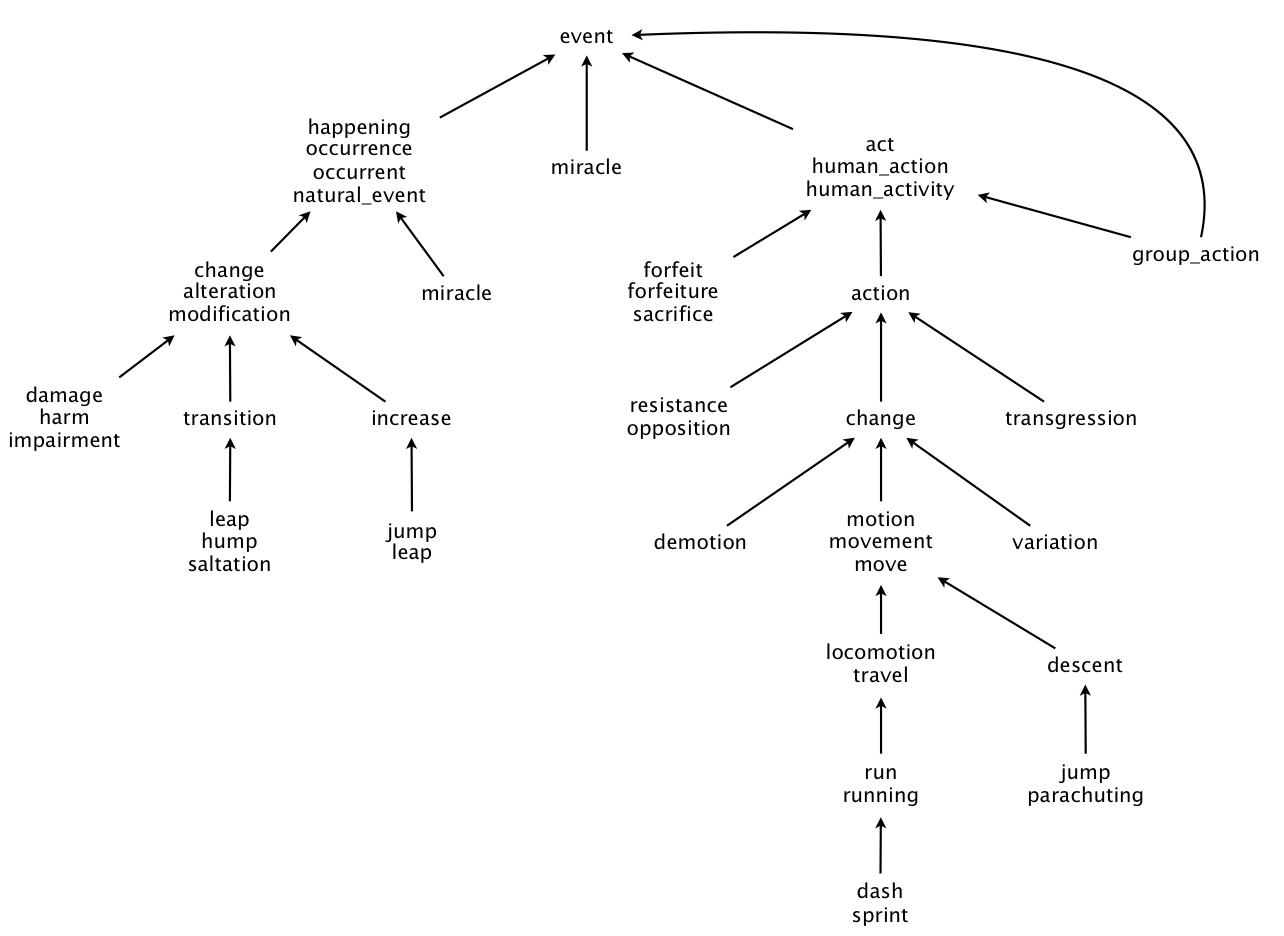The WordNet input file formats. We now describe the two data files that you will use to create the WordNet digraph. The files are in comma-separated values (CSV) format: each line contains a sequence of fields, separated by commas.

• List of synsets. The file synsets.txt contains all noun synsets in WordNet, one per line. Line i of the file (counting from 0) contains the information for synset i. The first field is the synset id, which is always the integer i; the second field is the synonym set (or synset); and the third field is its dictionary definition (or gloss), which is not relevant to this assignment.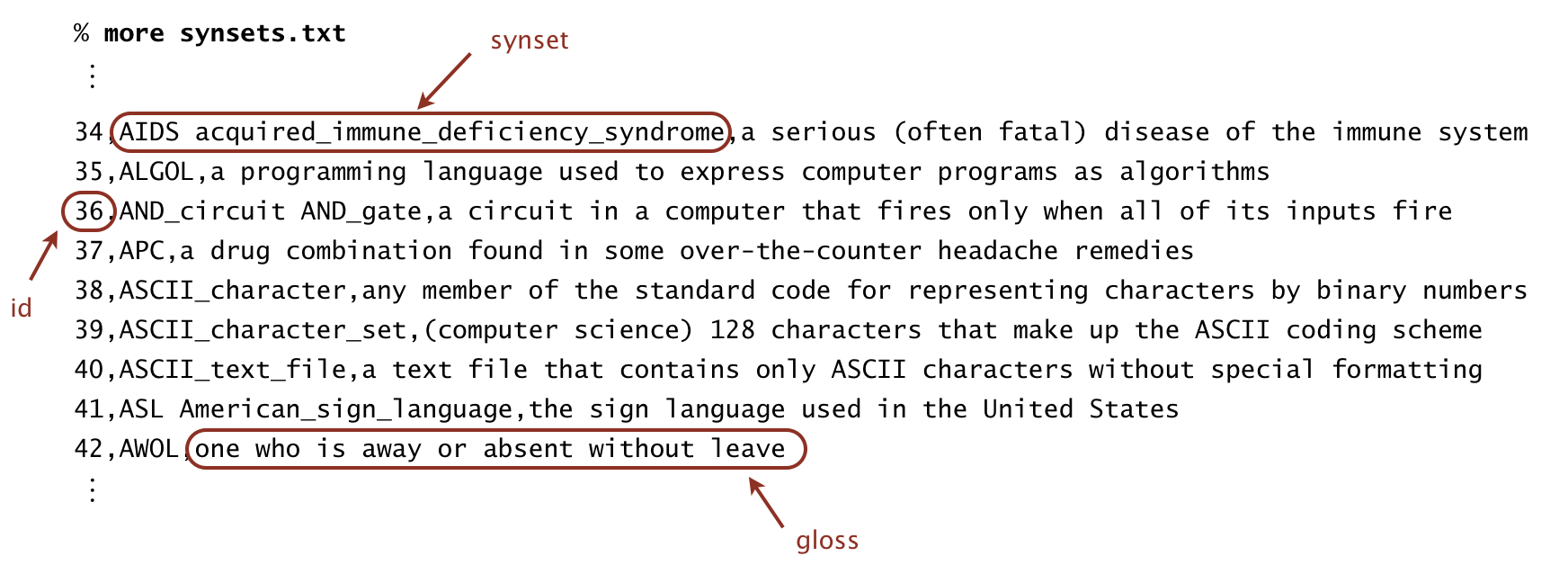For example, line 36 means that the synset { `AND_circuit`, `AND_gate` } has an id number of 36 and its gloss is `a circuit in a computer that fires only when all of its inputs fire`. The individual nouns that constitute a synset are separated by spaces. If a noun contains more than one word, the underscore character connects the words (and not the space character).
• List of hypernyms. The file hypernyms.txt contains the hypernym relationships. Line i of the file (counting from 0) contains the hypernyms of synset i. The first field is the synset id, which is always the integer i; subsequent fields are the id numbers of the synset’s hypernyms.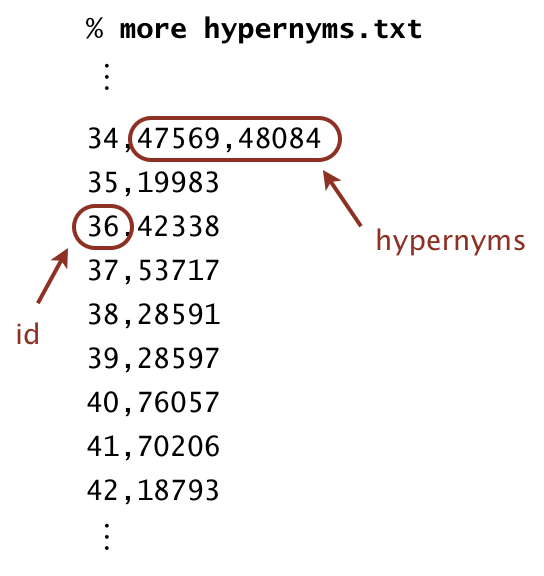For example, line 36 means that synset 36 (`AND_circuit AND_Gate`) has 42338 (`gate logic_gate`) as its only hypernym. Line 34 means that synset 34 (`AIDS acquired_immune_deficiency_syndrome`) has two hypernyms: 47569 (`immunodeficiency`) and 56099 (`infectious_disease`).

WordNet data type. Implement an immutable data type `WordNet` with the following API:

```public class WordNet {

// constructor takes the name of the two input files
public WordNet(String synsets, String hypernyms)

// all WordNet nouns
public Iterable<String> nouns()

// is the word a WordNet noun?
public boolean isNoun(String word)

// a synset (second field of synsets.txt) that is a shortest common ancestor
// of noun1 and noun2 (defined below)
public String sca(String noun1, String noun2)

// distance between noun1 and noun2 (defined below)
public int distance(String noun1, String noun2)

// unit testing (required)
public static void main(String[] args)
}
```
Corner cases.  Throw a `java.lang.IllegalArgumentException` in the following situations:
• Any argument to the constructor or an instance method is `null`
• Any of the noun arguments in `distance()` or `sca()` is not a WordNet noun.
You may assume that the input files are in the specified format and that the underlying digraph is a rooted DAG.

Unit testing.  Your `main()` method must call each public constructor and method directly and help verify that they work as prescribed (e.g., by printing results to standard output).

Performance requirements.  In the requirements below, assume that the number of characters in a noun or synset is bounded by a constant.

• Your data type must use space linear in the input size (size of synsets and hypernyms files).
• The constructor must take time linearithmic (or better) in the input size.
• The method `isNoun()` must run in time logarithmic (or better) in the number of nouns.
• The methods `distance()` and `sca()` must make exactly one call to the `lengthSubset()` and `ancestorSubset()` methods in `ShortestCommonAncestor`, respectively.

Shortest common ancestor. An ancestral path between two vertices v and w in a rooted DAG is a directed path from v to a common ancestor x, together with a directed path from w to the same ancestor x. A shortest ancestral path is an ancestral path of minimum total length. We refer to the common ancestor in a shortest ancestral path as a shortest common ancestor. Note that a shortest common ancestor always exists because the root is an ancestor of every vertex. Note also that an ancestral path is a path, but not a directed path.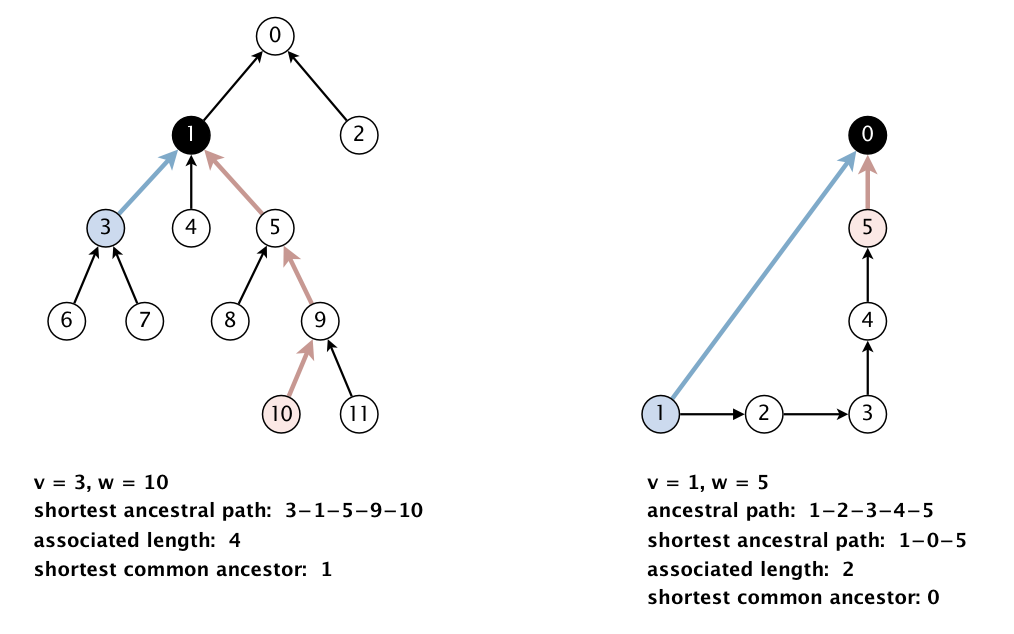We generalize the notion of shortest common ancestor to subsets of vertices. A shortest ancestral path of two subsets of vertices A and B is a shortest ancestral path over all pairs of vertices v and w, with v in A and w in B. The figure (digraph25.txt) below shows an example in which, for two subsets, red and blue, we have computed several (but not all) ancestral paths, including the shortest one.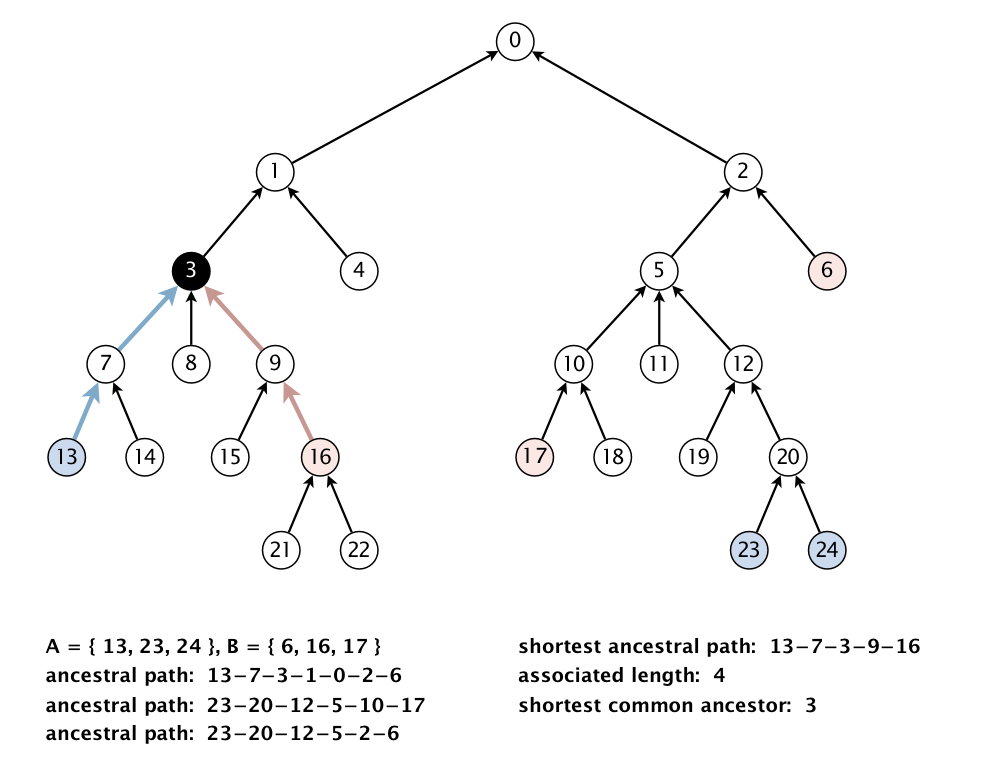Shortest common ancestor data type. Implement an immutable data type `ShortestCommonAncestor` with the following API:

```public class ShortestCommonAncestor {

// constructor takes a rooted DAG as argument
public ShortestCommonAncestor(Digraph G)

// length of shortest ancestral path between v and w
public int length(int v, int w)

// a shortest common ancestor of vertices v and w
public int ancestor(int v, int w)

// length of shortest ancestral path of vertex subsets A and B
public int lengthSubset(Iterable<Integer> subsetA, Iterable<Integer> subsetB)

// a shortest common ancestor of vertex subsets A and B
public int ancestorSubset(Iterable<Integer> subsetA, Iterable<Integer> subsetB)

// unit testing (required)
public static void main(String[] args)
}

```

Corner cases.  Throw a `java.lang.IllegalArgumentException` in the following situations:

• The argument to the constructor is not a rooted DAG
• Any argument is `null`
• Any vertex argument is outside its prescribed range
• Any iterable argument contains zero vertices
• Any iterable argument contains a `null` item

Unit testing.  Your `main()` method must call each public constructor and method directly and help verify that they work as prescribed (e.g., by printing results to standard output).

Basic performance requirements.  Your data type must use space proportional to E + V, where E and V are the number of edges and vertices in the digraph, respectively. All methods and the constructor must take time proportional to E + V (or better).

Additional performance requirements (for extra credit).  In addition, the methods `length()`, `lengthSubset()`, `ancestor()`, and `ancestorSubset()` must take time proportional to the number of vertices and edges reachable from the argument vertices (or better), For example, to compute the shortest common ancestor of v and w in the digraph below, your algorithm can examine only the highlighted vertices and edges; it cannot initialize any vertex-indexed arrays.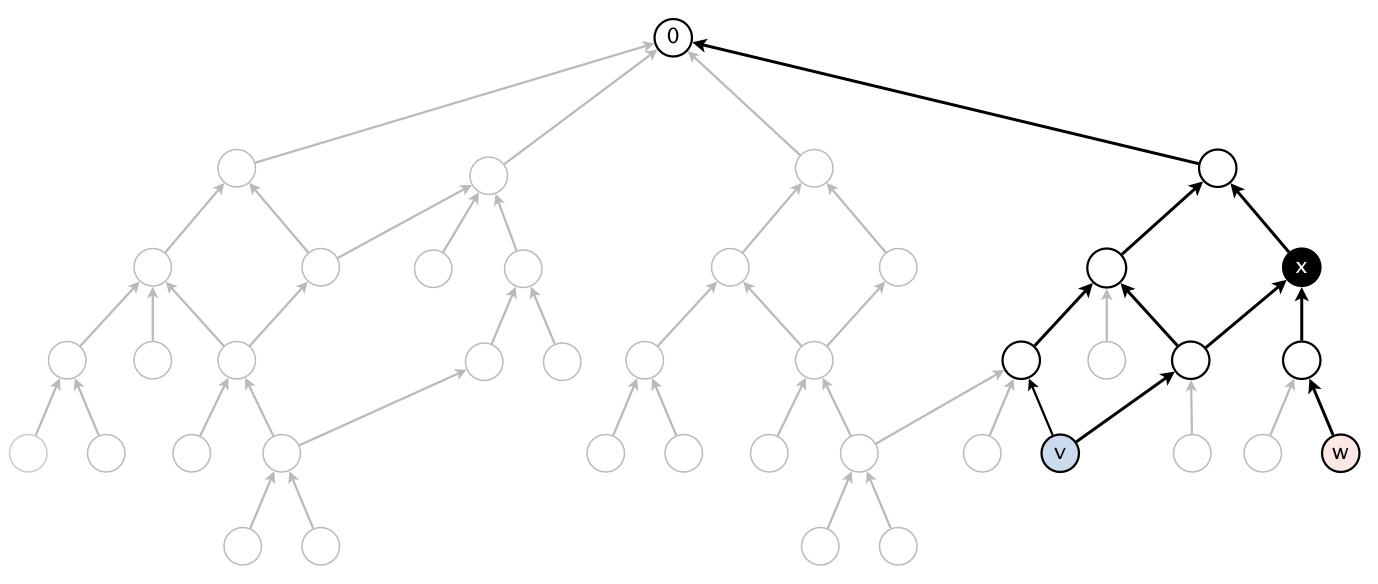Test client. The following test client takes the name of a digraph input file as as a command-line argument; creates the digraph; reads vertex pairs from standard input; and prints the length of the shortest ancestral path between the two vertices, along with a shortest common ancestor:

```public static void main(String[] args) {
In in = new In(args);
Digraph G = new Digraph(in);
ShortestCommonAncestor sca = new ShortestCommonAncestor(G);
while (!StdIn.isEmpty()) {
int length   = sca.length(v, w);
int ancestor = sca.ancestor(v, w);
StdOut.printf("length = %d, ancestor = %d\n", length, ancestor);
}
}
```
Here is a sample execution (the bold indicates what you type):
```% more digraph1.txt             % java-algs4 ShortestCommonAncestor digraph1.txt
12                              3 10
11                              length = 4, ancestor = 1
6  3
7  3                           8 11
3  1                           length = 3, ancestor = 5
4  1
5  1                           6 2
8  5                           length = 4, ancestor = 0
9  5
10  9
11  9
1  0
2  0
```

Measuring the semantic relatedness of two nouns. Semantic relatedness refers to the degree to which two concepts are related. Measuring semantic relatedness is a challenging problem. For example, you consider George W. Bush and John F. Kennedy (two U.S. presidents) to be more closely related than George W. Bush and chimpanzee (two primates). It might not be clear whether George W. Bush and Eric Arthur Blair are more related than two arbitrary people. However, both George W. Bush and Eric Arthur Blair (a.k.a. George Orwell) are famous communicators and, therefore, closely related.

We define the semantic relatedness of two WordNet nouns x and y as follows:

• A = set of synsets in which x appears

• B = set of synsets in which y appears

• distance(x, y) = length of shortest ancestral path of subsets A and B

• sca(x, y) = a shortest common ancestor of subsets A and B

This is the notion of distance that you will use to implement the `distance()` and `sca()` methods in the `WordNet` data type.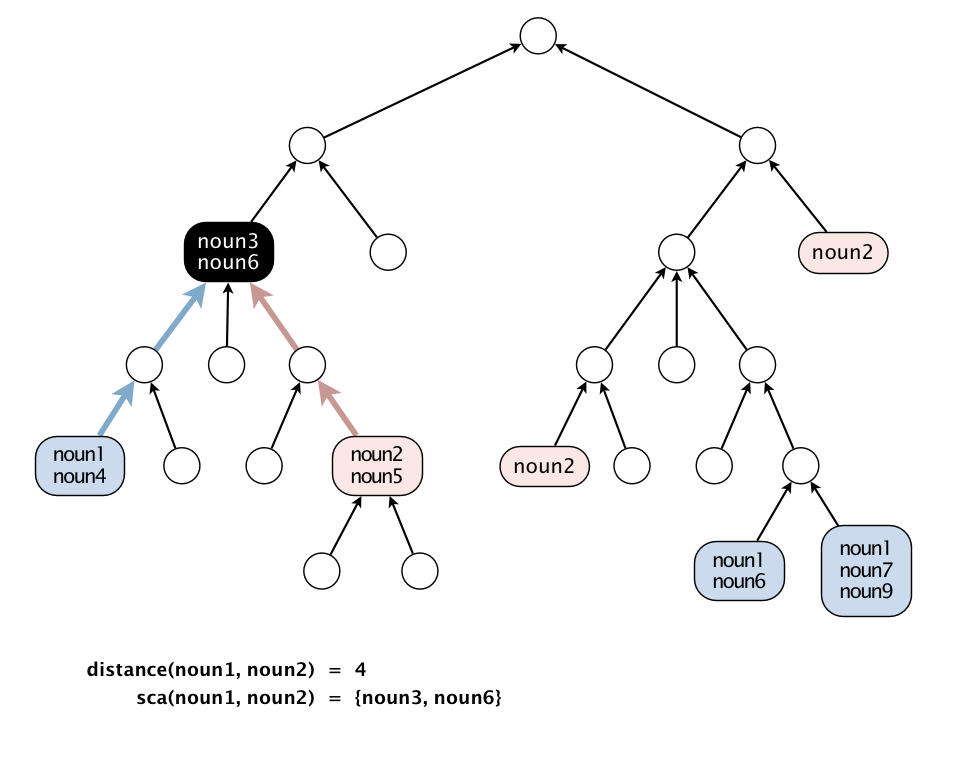Outcast detection. Given a list of WordNet nouns x1, x2, ..., xn, which noun is the least related to the others? To identify an outcast, compute the sum of the distances between each noun and every other one:

di   =   distance(xi, x1)   +   distance(xi, x2)   +   ...   +   distance(xi, xn)
and return a noun xt for which dt is maximum. Note that distance(xi, xi) = 0, so it will not contribute to the sum.

Implement an immutable data type `Outcast` with the following API:

```public class Outcast {
public Outcast(WordNet wordnet)         // constructor takes a WordNet object
public String outcast(String[] nouns)   // given an array of WordNet nouns, return an outcast

public static void main(String[] args)  // test client (see below)
}
```

Corner cases. Assume that the argument to `outcast()` contains only valid WordNet nouns and that it contains at least two such nouns.

Test client. The following test client takes from the command line the name of a synset file, the name of a hypernym file, followed by the names of outcast files, and prints an outcast in each file:

```public static void main(String[] args) {
WordNet wordnet = new WordNet(args, args);
Outcast outcast = new Outcast(wordnet);
for (int t = 2; t < args.length; t++) {
In in = new In(args[t]);
StdOut.println(args[t] + ": " + outcast.outcast(nouns));
}
}
```
Here is a sample execution:
```% more outcast5.txt
horse zebra cat bear table

% more outcast8.txt
water soda bed orange_juice milk apple_juice tea coffee

% more outcast11.txt
apple pear peach banana lime lemon blueberry strawberry mango watermelon potato

% java-algs4 Outcast synsets.txt hypernyms.txt outcast5.txt outcast8.txt outcast11.txt
outcast5.txt: table
outcast8.txt: bed
outcast11.txt: potato
```

Analysis of running time. Analyze the potential effectiveness of your approach to this problem by answering the following questions:

• What is the order of growth of the worst-case running time of the `length()`, `lengthAncestor()`, `ancestor()`, and `ancestorSubset()` methods in `ShortestCommonAncestor`?

• What is the order of growth of the best-case running time of the `length()`, `lengthAncestor()`, `ancestor()`, and `ancestorSubset()` methods in `ShortestCommonAncestor`?
Give your answers as a function of the number of vertices V and the number of edges E in the digraph.

Deliverables. Submit `WordNet.java`, `ShortestCommonAncestor.java`, and `Outcast.java` that implement the APIs described above, along with a readme.txt file. Also submit any other supporting files (excluding those in `algs4.jar`). You may not call any library functions other than those in `java.lang`, `java.util`, and `algs4.jar`.

This assignment was created by Alina Ene and Kevin Wayne.